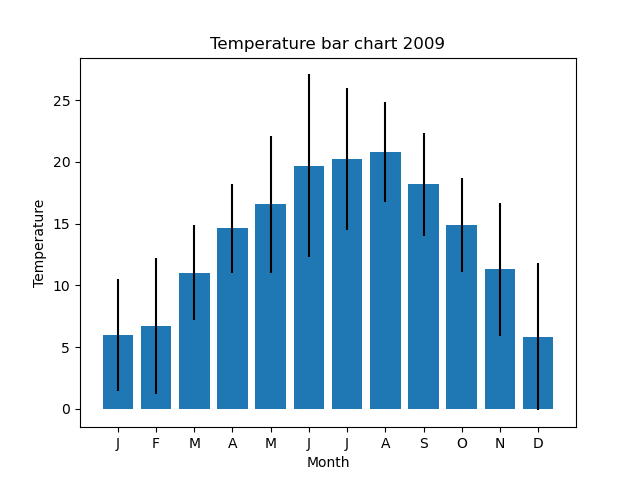# Error bars in Matplotlib

By Martin McBride, 2022-06-23
Tags: matplotlib bar chart error bars
Categories: matplotlibWe can add error bars to our chart, to indicate the degree of variation in the data. Here is what it looks like:Here is the code to plot this chart:

import matplotlib.pyplot as plt
import csv

with open("2009-temp-monthly-list.csv") as csv_file:

month_names = ["J", "F", "M", "A", "M", "J",
"J", "A", "S", "O", "N", "D"]

months = range(12)

temperature = [sum(k)/len(k) for k in temperature_lists]
errors = [(max(k)-min(k))/2 for k in temperature_lists]

plt.title("Temperature bar chart 2009")
plt.xlabel("Month")
plt.ylabel("Temperature")
plt.bar(months, temperature, yerr=errors)
plt.xticks(months, month_names)
plt.show()


This code is available on github as barchart_error_bars.py.

We will look at the important new features used in this code.

## The data

Since we want to plot tha variance of the data, we cannot use the 2009-temp-monthly.csv data. That data only contains one value for each month, the average value.

Instead, we use the 2009-temp-monthly-list.csv data. This is a list of 12 sublists. Each sublist contains the temperatures of each day in the month. The first sublist contains 31 values corresponding to the temperatures of the 31 days in January. Yje second contains 28 values for the days in February, and so on. This is read into temperature_lists in the code above.

See the description of the data sets.

## Processing the data

We need to calculate 2 sets of values:

• The average temperature for each month. This creates a list temperature of 12 values that determine the height of each bar in the graph.
• The error range of temperatures for each month. This creates a list errors of 12 values that determine the length of each error bar.

Here are the calculations:

temperature = [sum(k)/len(k) for k in temperature_lists]
errors = [(max(k)-min(k))/2 for k in temperature_lists]


To calculate the average temperature, we loop over every sublist k. We divide the sum of the elements in the sublist (given by sum(k)) by the number of the elements in the sublist (given by len(k)), which of course tells us the mean average. This will be the same value that is present in the 2009-temp-monthly.csv data.

We also calculate the error range errors for each month. There are various ways to calculate this, and you can use whichever one you prefer. To keep things simple, we just take the difference between the maximum value and the minimum value, divided by 2.

## Plotting the error bars

Here is how we plot the error bars:

plt.bar(months, temperature, yerr=errors)


We simply pass in the errors list as the yerr parameter.